# The MI Procedure

### TRANSFORM Statement

• TRANSFORM transform (variables</ options>)<…transform (variables</ options>)> ;

The TRANSFORM statement lists the transformations and their associated variables to be transformed. The options are transformation options that provide additional information for the transformation.

The MI procedure assumes that the data are from a multivariate normal distribution when either the regression method or the MCMC method is used. When some variables in a data set are clearly non-normal, it is useful to transform these variables to conform to the multivariate normality assumption. With a TRANSFORM statement, variables are transformed before the imputation process, and these transformed variable values are displayed in all of the results. When you specify an OUT= option, the variable values are back-transformed to create the imputed data set.

The following transformations can be used in the TRANSFORM statement:

BOXCOX

specifies the Box-Cox transformation of variables. The variable Y is transformed to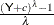, where c is a constant such that each value of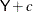must be positive. If the specified constant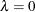, the logarithmic transformation is used.

EXP

specifies the exponential transformation of variables. The variable Y is transformed to, where c is a constant.

LOG

specifies the logarithmic transformation of variables. The variable Y is transformed to, where c is a constant such that each value ofmust be positive.

LOGIT

specifies the logit transformation of variables. The variable Y is transformed to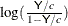, where the constant c>0 and the values of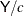must be between 0 and 1.

POWER

specifies the power transformation of variables. The variable Y is transformed to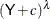, where c is a constant such that each value ofmust be positive and the constant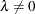.

The following options provide the constant c andvalues in the transformations.

C=number

specifies the c value in the transformation. The default is c = 1 for logit transformation and c = 0 for other transformations.

LAMBDA=number

specifies thevalue in the power and Box-Cox transformations. You must specify thevalue for these two transformations.

For example, the following statement requests that variables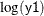, a logarithmic transformation for the variable y1, and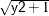, a power transformation for the variable y2, be used in the imputation:

   transform log(y1) power(y2/c=1 lambda=.5);


If the MU0= option is used to specify a parameter valuefor a transformed variable, the same transformation for the variable is also applied to its corresponding MU0= value in the t test. Otherwise,is used for the transformed variable. See Example 63.10 for a usage of the TRANSFORM statement.